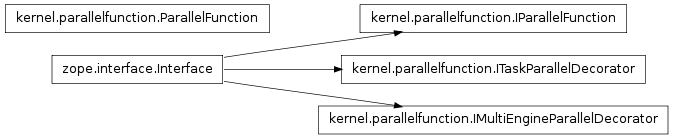# kernel.parallelfunction¶

## Module: kernel.parallelfunction¶

Inheritance diagram for IPython.kernel.parallelfunction:A parallelized function that does scatter/execute/gather.

## Classes¶

### IMultiEngineParallelDecorator¶

class IPython.kernel.parallelfunction.IMultiEngineParallelDecorator(name, bases=(), attrs=None, __doc__=None, __module__=None)

Bases: zope.interface.Interface

A decorator that creates a parallel function.

static __init__(name, bases=(), attrs=None, __doc__=None, __module__=None)

### IParallelFunction¶

class IPython.kernel.parallelfunction.IParallelFunction(name, bases=(), attrs=None, __doc__=None, __module__=None)

Bases: zope.interface.Interface

static __init__(name, bases=(), attrs=None, __doc__=None, __module__=None)

class IPython.kernel.parallelfunction.ITaskParallelDecorator(name, bases=(), attrs=None, __doc__=None, __module__=None)

Bases: zope.interface.Interface

A decorator that creates a parallel function.

static __init__(name, bases=(), attrs=None, __doc__=None, __module__=None)

### ParallelFunction¶

class IPython.kernel.parallelfunction.ParallelFunction(mapper)

Bases: object

The implementation of a parallel function.

A parallel function is similar to Python’s map function:

map(func, *sequences) -> pfunc(*sequences)

Parallel functions should be created by using the @parallel decorator.

__init__(mapper)

Create a parallel function from an IMapper.

Parameters: mapper : an IMapper implementer. The mapper to use for the parallel function